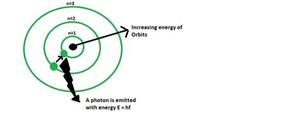Open in App
Not now

# Bohr’s Atomic Model

• Difficulty Level : Medium
• Last Updated : 13 Oct, 2021

Bohr’s Model is an atomic model proposed by a Danish Physicist Niels Bohr in 1913. According to this model, In an atom, the electrons revolve around the nucleus in definite energy levels called orbits/shells. The model proposes that the maximum number of electrons that can be accommodated in any particular orbit is 2n2  where n is the number of orbits. and the maximum number of electrons that can be accommodated in the outermost shell of an atom is 8.

Also, the orbit which is closest to the nucleus has the minimum energy, and the orbit farthest from the nucleus has maximum energy. Electrons are excited to higher energy levels by absorbing energy and returns to lower energy level by radiating energy.Bohr’s Atomic model

### Historical Background of Bohr’s Model

Bohr’s model came in form of an improvement after the previously proposed Atomic models like the cubic model (1902), plum-pudding model (1904), Saturnian model (1904), and Rutherford model (1911). Bohr model is mainly the modification based on quantum-physics over the Rutherford model, which was based on classical physics.

The model was able to successfully explain the concept of the Rydberg formula for the spectral emission lines of atomic hydrogen. Previously the Rydberg formula was known only experimentally, but theoretically, it was not proved, the Bohr model introduced the theoretical concept successfully.

The previously proposed planetary models of the atom also had some problems. They used to consider electrons spinning in orbit around a nucleus as a charged particle moving in an electric field, but there was no explanation of the fact that the electron would spiral into the nucleus. Viewing in terms of electron emission, this would represent a continuum of frequencies being emitted since, as the electron moved closer to the nucleus, it will move faster and will emit a different frequency which was observed experimentally. These planetary models ultimately predicted all atoms to be unstable due to the orbital decay, which was not true and the phenomenon was successfully explained by Bohr’s model.

### Postulates of Bohr’s atomic model

First Postulate: In an atom, electrons revolve around the nucleus in some fixed circular orbits (called stationary orbits), electrons don’t emit energy which was expected from electrodynamics.

Second Postulate: Stationary orbits are those orbits in which the angular momentum of the electron is the integral angular momentum of the electron is an integral multiple of “h/2π”, where “h” is the Planck’s constant.

Therefore the angular momentum “L” of the revolving electron is quantized.

Mathematically,

L = mvr = nh/2π

where, n is integer 1,2,3….. known as a principal quantum number.

Third Postulate: The energy of an electron revolving in a stationary orbit remains constant. Energy is emitted from an atom only when an electron jumps from the orbit of higher energy to the orbit having low energy.

When it happens, a photon is limited whose energy is equal to the difference of energy of two orbits. If Ei and Ej are the energies of orbits in the initial and final situation, the frequency of the photon emitted will be given by the following equation.

hv=Ei-Ef

An electron may jump in a higher energy orbit by absorbing energy from outside. In this condition, the energy of photons absorbed is.

hv=Ef-Ei

### Limitations of Bohr’s Atomic Model

1. The model was applicable to uni electron system only
2. The model couldn’t explain the presence of fine line structures of different spectral lines of the hydrogen spectrum
3. The model couldn’t explain the splitting of spectral lines in the presence of magnetic fields ( Zeeman’s effect  ), and electric fields (Stark effect)

### Sample Questions

Question 1: If the velocity of an electron of the first orbit in Bohr’s atomic model of a hydrogen atom is 2.19 × 106 m/s. Find the velocity of electrons in the second orbit.

The velocity of an electron in orbit n is given by  vn=v1/n

So, v2 = 2.19 × 106 m/s / 2

= 1.095 × 106 m/s.

Question 2: The electron in Bohr’s Hydrogen atom, revolves around the nucleus with a speed of 2.18 × 106 m/s in orbit of radius 5.3 × 10-11 m. Find the magnetic field which is produced by electrons on protons in the nucleus.

Given,

Radius of orbit, r = 5.3 × 10-11 m

Velocity v= 2.18 × 106 m/s

So, magnetic field, I = Q/T

= e/T

where T = 2πr / v

= (2 × 3.14 × 5.3 × 10-11) / (2.18 × 106)

= 1.528 × 10-16

Therefore,

I = 1.6 × 10-19/ 1.528×10-16

= 1.047 × 10-3 A

Question 3: Find the kinetic energy of an electron in the second Bohr’s orbit of a hydrogen atom.

According to Bohr’s postulate

mvr = nh / 2π

v = nh / 2πmr

K .E = 1/2 mv2

= 1/2 m ( nh / 2πmr ) 2

Question 4: Find the distance between the 2nd and 3rd orbit of Bohr’s atom.

The radius of 3rd orbit r3 = 0.529 × n2 × 10-10 / z

= 0.529 × 9 × 10-10

The radius of 2nd orbit r2 = 0.529 × 4 × 10-10

r3 – r2 = 0.529 × (5 × 10 -10)m

= 2.645 A

Question 5: Find the distance between the 2nd and 3rd orbit of Bohr’s atom.

Theradius of 2nd orbit r3 = 0.529 × n2 × 10-10 / z

= 0.529 × 4 × 10-10

radius of 1st orbit r2 = 0.529 × 1 × 10-10

r2– r1 = 0.529 × ( 3 × 10-10)m

= 1.587 A

My Personal Notes arrow_drop_up